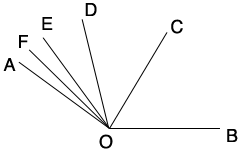SEARCH HOMEMath Central Quandaries & QueriesQuestion from frank, a parent: Ray OC bisects angle AOB, ray OD bisects angle AOC, ray OE bisects angle AOD, ray OF bisects angel AOE, and ray OG bisects angle FOC: if angle BOF=120 degrees, then find angle DOE? Confused!Frank,

I drew a diagram.The point G doesn't appear since it is unimportant is solving the problem.

Suppose the measure of the angle BOC is x degrees.
What is the measure of the angle COD?
What is the measure of the angle DOE?
What is the measure of the angle EOF?

The sum of these measures is 120 degrees. Solve for x.

I hope this helps,
PennyMath Central is supported by the University of Regina and The Pacific Institute for the Mathematical Sciences.